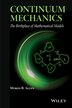# Continuum Mechanics: The Birthplace of Mathematical Models

## byMyron B. Allen

July 20, 2015|\$107.96
\$125.00
Hardcover
Earn 540 plum® points
Prices and offers may vary in store

Out of stock online

Not available in stores

Presents a self-contained introduction to continuum mechanics that illustrates how many of the important partial differential equations of applied mathematics arise from continuum modeling principles

Written as an accessible introduction, Continuum Mechanics: The Birthplace of Mathematical Models provides a comprehensive foundation for mathematical models used in fluid mechanics, solid mechanics, and heat transfer. The book features derivations of commonly used differential equations based on the fundamental continuum mechanical concepts encountered in various fields, such as engineering, physics, and geophysics.

The book begins with geometric, algebraic, and analytical foundations before introducing topics in kinematics. The book then addresses balance laws, constitutive relations, and constitutive theory. Finally, the book presents an approach to multiconstituent continua based on mixture theory to illustrate how phenomena, such as diffusion and porous-media flow, obey continuum-mechanical principles.

Continuum Mechanics: The Birthplace of Mathematical Models features:

• Direct vector and tensor notation to minimize the reliance on particular coordinate systems when presenting the theory
• Terminology that is aligned with standard courses in vector calculus and linear algebra
• The use of Cartesian coordinates in the examples and problems to provide readers with a familiar setting
• Over 200 exercises and problems with hints and solutions in an appendix
• Introductions to constitutive theory and multiconstituent continua, which are distinctive for books at this level
Continuum Mechanics: The Birthplace of Mathematical Models is an ideal textbook for courses on continuum mechanics for upper-undergraduate mathematics majors and graduate students in applied mathematics, mechanical engineering, civil engineering, physics, and geophysics. The book is also an excellent reference for professional mathematicians, physical scientists, and engineers.
Title:Continuum Mechanics: The Birthplace of Mathematical ModelsFormat:HardcoverProduct dimensions:288 pages, 9.5 X 6.5 X 1 inShipping dimensions:288 pages, 9.5 X 6.5 X 1 inPublished:July 20, 2015Publisher:WileyLanguage:English

The following ISBNs are associated with this title:

ISBN - 10:1118909372

ISBN - 13:9781118909379

Appropriate for ages: All ages

Look for similar items by category: